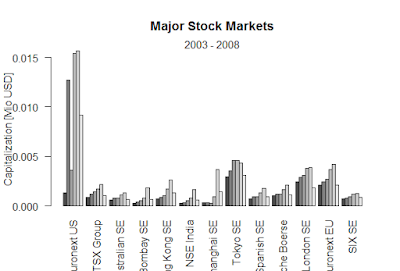Showing posts with label graphics code in R. Show all posts
Showing posts with label graphics code in R. Show all posts

## How to use graphics code in R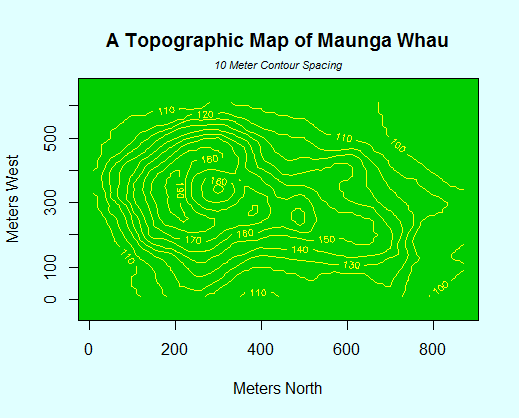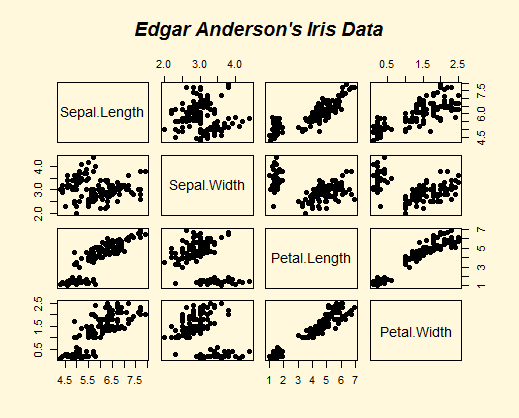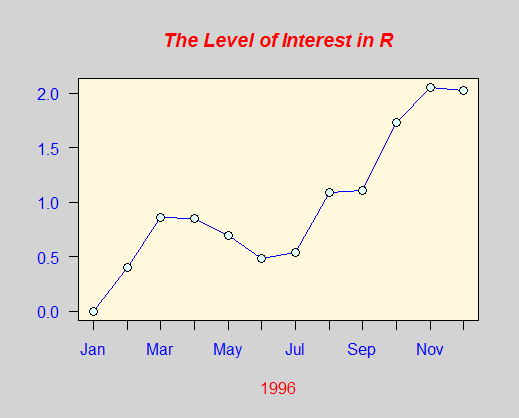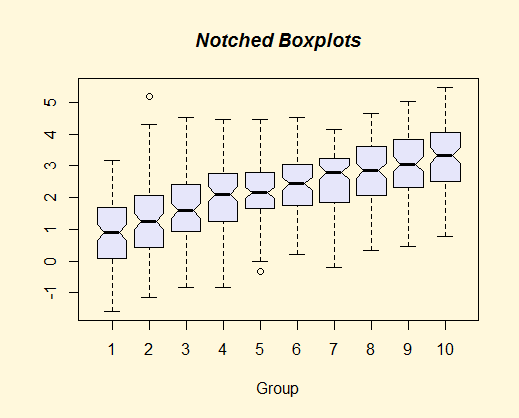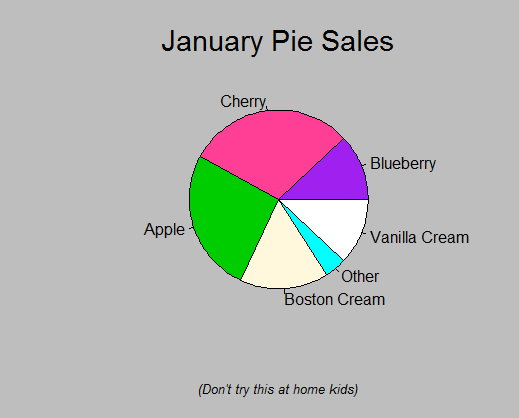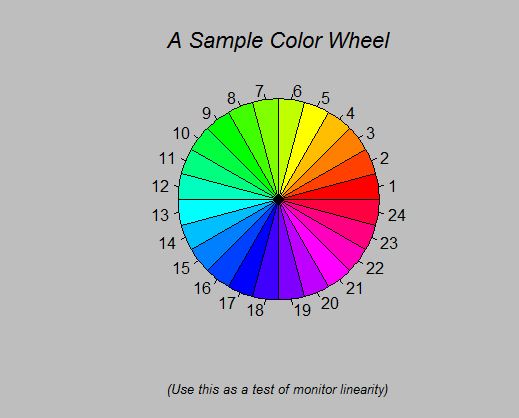demo(graphics)
---- ~~~~~~~~

Type  <Return> to start :

> #  Copyright (C) 1997-2009 The R Core Team
>
> require(datasets)

> require(grDevices); require(graphics)

> ## Here is some code which illustrates some of the differences between
> ## R and S graphics capabilities.  Note that colors are generally specified
> ## by a character string name (taken from the X11 rgb.txt file) and that line
> ## textures are given similarly.  The parameter "bg" sets the background
> ## parameter for the plot and there is also an "fg" parameter which sets
> ## the foreground color.
>
>
> x <- stats::rnorm(50)

> opar <- par(bg = "white")

> plot(x, ann = FALSE, type = "n")
Hit <Return> to see next plot:

> abline(h = 0, col = gray(.90))

> lines(x, col = "green4", lty = "dotted")

> points(x, bg = "limegreen", pch = 21)

> title(main = "Simple Use of Color In a Plot",
+       xlab = "Just a Whisper of a Label",
+       col.main = "blue", col.lab = gray(.8),
+       cex.main = 1.2, cex.lab = 1.0, font.main = 4, font.lab = 3)

> ## A little color wheel. This code just plots equally spaced hues in
> ## a pie chart. If you have a cheap SVGA monitor (like me) you will
> ## probably find that numerically equispaced does not mean visually
> ## equispaced.  On my display at home, these colors tend to cluster at
> ## the RGB primaries.  On the other hand on the SGI Indy at work the
> ## effect is near perfect.
>
> par(bg = "gray")

> pie(rep(1,24), col = rainbow(24), radius = 0.9)
Hit <Return> to see next plot:

> title(main = "A Sample Color Wheel", cex.main = 1.4, font.main = 3)

> title(xlab = "(Use this as a test of monitor linearity)",
+       cex.lab = 0.8, font.lab = 3)

> ## We have already confessed to having these.  This is just showing off X11
> ## color names (and the example (from the postscript manual) is pretty "cute".
>
> pie.sales <- c(0.12, 0.3, 0.26, 0.16, 0.04, 0.12)

> names(pie.sales) <- c("Blueberry", "Cherry",
+       "Apple", "Boston Cream", "Other", "Vanilla Cream")

> pie(pie.sales,
+     col = c("purple","violetred1","green3","cornsilk","cyan","white"))
Hit <Return> to see next plot:

> title(main = "January Pie Sales", cex.main = 1.8, font.main = 1)

> title(xlab = "(Don't try this at home kids)", cex.lab = 0.8, font.lab = 3)

> ## Boxplots:  I couldn't resist the capability for filling the "box".
> ## The use of color seems like a useful addition, it focuses attention
> ## on the central bulk of the data.
>
> par(bg="cornsilk")

> n <- 10

> g <- gl(n, 100, n*100)

> x <- rnorm(n*100) + sqrt(as.numeric(g))

> boxplot(split(x,g), col="lavender", notch=TRUE)
Hit <Return> to see next plot:

> title(main="Notched Boxplots", xlab="Group", font.main=4, font.lab=1)

> ## An example showing how to fill between curves.
>
> par(bg="white")

> n <- 100

> x <- c(0,cumsum(rnorm(n)))

> y <- c(0,cumsum(rnorm(n)))

> xx <- c(0:n, n:0)

> yy <- c(x, rev(y))

> plot(xx, yy, type="n", xlab="Time", ylab="Distance")
Hit <Return> to see next plot:

> polygon(xx, yy, col="gray")

> title("Distance Between Brownian Motions")

> ## Colored plot margins, axis labels and titles. You do need to be
> ## careful with these kinds of effects. It's easy to go completely
> ## over the top and you can end up with your lunch all over the keyboard.
> ## On the other hand, my market research clients love it.
>
> x <- c(0.00, 0.40, 0.86, 0.85, 0.69, 0.48, 0.54, 1.09, 1.11, 1.73, 2.05, 2.02)

> par(bg="lightgray")

> plot(x, type="n", axes=FALSE, ann=FALSE)
Hit <Return> to see next plot:

> usr <- par("usr")

> rect(usr, usr, usr, usr, col="cornsilk", border="black")

> lines(x, col="blue")

> points(x, pch=21, bg="lightcyan", cex=1.25)

> axis(2, col.axis="blue", las=1)

> axis(1, at=1:12, lab=month.abb, col.axis="blue")

> box()

> title(main= "The Level of Interest in R", font.main=4, col.main="red")

> title(xlab= "1996", col.lab="red")

> ## A filled histogram, showing how to change the font used for the
> ## main title without changing the other annotation.
>
> par(bg="cornsilk")

> x <- rnorm(1000)

> hist(x, xlim=range(-4, 4, x), col="lavender", main="")
Hit <Return> to see next plot:

> title(main="1000 Normal Random Variates", font.main=3)

> ## A scatterplot matrix
> ## The good old Iris data (yet again)
>
> pairs(iris[1:4], main="Edgar Anderson's Iris Data", font.main=4, pch=19)
Hit <Return> to see next plot:

> pairs(iris[1:4], main="Edgar Anderson's Iris Data", pch=21,
+       bg = c("red", "green3", "blue")[unclass(iris\$Species)])
Hit <Return> to see next plot:

> ## Contour plotting
> ## This produces a topographic map of one of Auckland's many volcanic "peaks".
>
> x <- 10*1:nrow(volcano)

> y <- 10*1:ncol(volcano)

> lev <- pretty(range(volcano), 10)

> par(bg = "lightcyan")

> pin <- par("pin")

> xdelta <- diff(range(x))

> ydelta <- diff(range(y))

> xscale <- pin/xdelta

> yscale <- pin/ydelta

> scale <- min(xscale, yscale)

> xadd <- 0.5*(pin/scale - xdelta)

> yadd <- 0.5*(pin/scale - ydelta)

> plot(numeric(0), numeric(0),
+      type = "n", ann = FALSE)
Hit <Return> to see next plot:

> usr <- par("usr")

> rect(usr, usr, usr, usr, col="green3")

> contour(x, y, volcano, levels = lev, col="yellow", lty="solid", add=TRUE)

> box()

> title("A Topographic Map of Maunga Whau", font= 4)

> title(xlab = "Meters North", ylab = "Meters West", font= 3)

> mtext("10 Meter Contour Spacing", side=3, line=0.35, outer=FALSE,
+       at = mean(par("usr")[1:2]), cex=0.7, font=3)

> ## Conditioning plots
>
> par(bg="cornsilk")

> coplot(lat ~ long | depth, data = quakes, pch = 21, bg = "green3")
Hit <Return> to see next plot:

> par(opar)

### Black-Scholes formula-R

Black-Scholes formula-R > BlackScholes <- function(TypeFlag = c("c", "p"), S, X, Time, r, b, sigma) { TypeFla...